2019/03/14 23:47

# 结对作业博客

### PSP表格估计时间

PSP2.1 Personal Software Process Stages 预估耗时（分钟） 实际耗时（分钟）
Planning 计划
· Estimate · 估计这个任务需要多少时间 10 30
Development 开发
· Analysis · 需求分析 (包括学习新技术) 45 60
· Design Spec · 生成设计文档 45 45
· Design Review · 设计复审 (和同事审核设计文档) 45 45
· Coding Standard · 代码规范 (为目前的开发制定合适的规范) 45 45
· Design · 具体设计 60 90
· Coding · 具体编码 1000 1500
· Code Review · 代码复审 60 120
· Test · 测试（自我测试，修改代码，提交修改） 360 800
Reporting 报告
· Test Report · 测试报告 60 60
· Size Measurement · 计算工作量 30 30
· Postmortem & Process Improvement Plan · 事后总结, 并提出过程改进计划 30 30

### 计算模块接口的设计与实现过程

​ 最重要的函数就是find_Longest_Chain()函数和find_Longest_Chain_With_R()函数。对于find_Longest_Chain()函数，由于没有环路，因此每个字母实际上只需要计算一次，如果发现这个字母已经计算过了，就直接返回它的最长链的长度。如果没计算过，就判断它是不是到达了结尾。如果是结尾，根据可能规定的结尾字母来判断这个字母的最长链长度。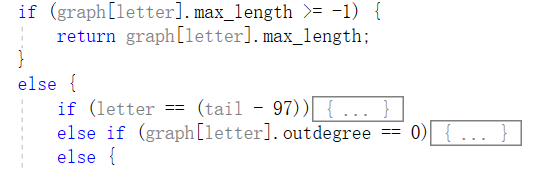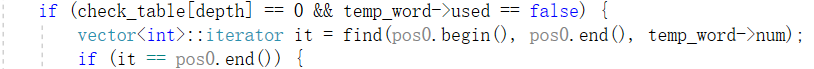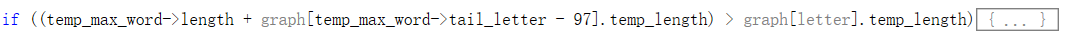### 计算模块接口部分的性能改进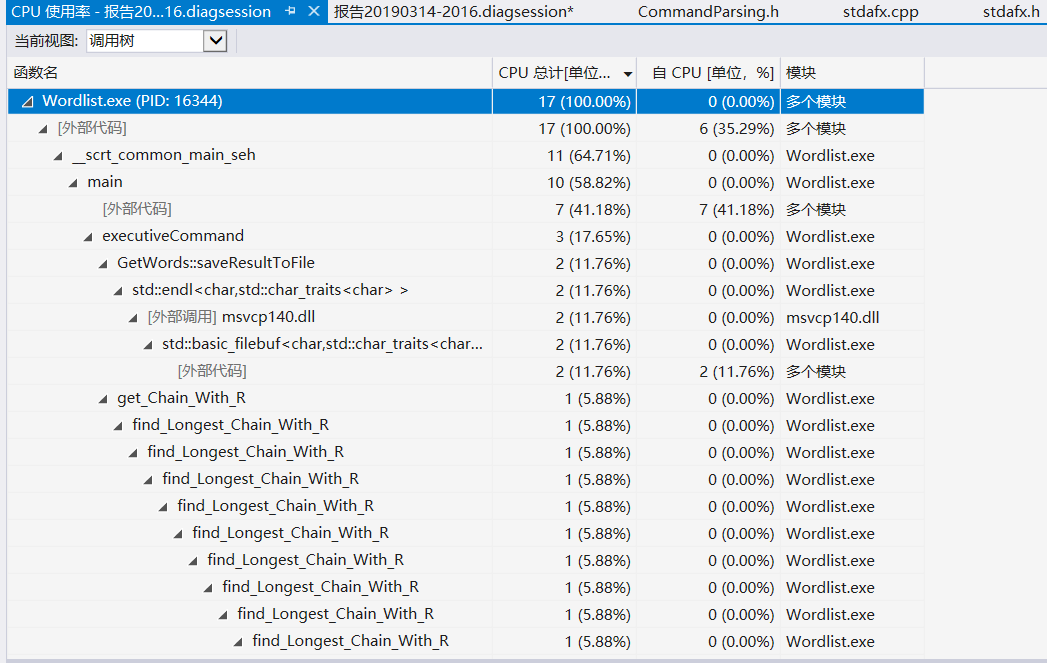### 看Design by Contract, Code Contract的内容，描述这些做法的优缺点, 说明你是如何把它们融入结对作业中的

Design by Contract的优点 首先优点就是能够有一个规范，这样子对于大型团队而言，人员众多，能够减少代码中的风格差异，还有接口，这样能够让这种大型项目比较融洽的进行下去。不至于最后又要统一各种各样的接口、函数而浪费时间。 Design by Contract的缺点 其最主要的缺点在于，如果要制定详细的规范，会花费大量的时间，尤其是在时间较为紧迫的情况下，不得不去稍微放款点标准，毕竟不可能在一开始就想到某些接口的具体实现，这个需要一定时间的判断。最终拖累了项目。而在人员较少的时候，当面沟通比较方便的时候，可以在撰写的同时约定好，不用事先进行约定。

### 计算模块部分单元测试展示

TEST_METHOD(RightTest)
{
char * argv_temp;
int argc_temp = 7;

ofstream outputFile("temp.txt");
outputFile << "aaa abb bbb bcc ccc cdd";
outputFile.close();

argv_temp = (char*)malloc(sizeof(char) * 14);
strcpy(argv_temp, "WordList.exe");
argv_temp = (char*)malloc(sizeof(char) * 3);
strcpy(argv_temp, "-w");
argv_temp = (char*)malloc(sizeof(char) * 3);
strcpy(argv_temp, "-h");
argv_temp = (char*)malloc(sizeof(char) * 2);
strcpy(argv_temp, "a");
argv_temp = (char*)malloc(sizeof(char) * 3);
strcpy(argv_temp, "-t");
argv_temp = (char*)malloc(sizeof(char) * 2);
strcpy(argv_temp, "c");
argv_temp = (char*)malloc(sizeof(char) * 10);
strcpy(argv_temp, "temp.txt");

string error_message;
Assert::AreEqual((double)kErrorNone,
(double)executiveCommand(argc_temp, argv_temp, true, &error_message));
Assert::AreEqual(error_message.c_str(),
"");
}


TEST_METHOD(RightTestWithR)
{
char * argv_temp;
int argc_temp = 8;

ofstream outputFile("temp.txt");
outputFile << "aaa abb acc baa bbb bcc caa cbb ccc";
outputFile.close();

argv_temp = (char*)malloc(sizeof(char) * 14);
strcpy(argv_temp, "WordList.exe");
argv_temp = (char*)malloc(sizeof(char) * 3);
strcpy(argv_temp, "-w");
argv_temp = (char*)malloc(sizeof(char) * 3);
strcpy(argv_temp, "-h");
argv_temp = (char*)malloc(sizeof(char) * 2);
strcpy(argv_temp, "a");
argv_temp = (char*)malloc(sizeof(char) * 3);
strcpy(argv_temp, "-t");
argv_temp = (char*)malloc(sizeof(char) * 2);
strcpy(argv_temp, "c");
argv_temp = (char*)malloc(sizeof(char) * 3);
strcpy(argv_temp, "-r");
argv_temp = (char*)malloc(sizeof(char) * 10);
strcpy(argv_temp, "temp.txt");

string error_message;
Assert::AreEqual((double)kErrorNone,
(double)executiveCommand(argc_temp, argv_temp, true, &error_message));
Assert::AreEqual(error_message.c_str(),
"");
}


TEST_METHOD(ErrorCirculation)
{
char * argv_temp;
int argc_temp = 7;

ofstream outputFile("temp.txt");
outputFile << "aaa abb acc baa bbb bcc caa cbb ccc";
outputFile.close();

argv_temp = (char*)malloc(sizeof(char) * 14);
strcpy(argv_temp, "WordList.exe");
argv_temp = (char*)malloc(sizeof(char) * 3);
strcpy(argv_temp, "-w");
argv_temp = (char*)malloc(sizeof(char) * 3);
strcpy(argv_temp, "-h");
argv_temp = (char*)malloc(sizeof(char) * 2);
strcpy(argv_temp, "a");
argv_temp = (char*)malloc(sizeof(char) * 3);
strcpy(argv_temp, "-t");
argv_temp = (char*)malloc(sizeof(char) * 2);
strcpy(argv_temp, "c");
argv_temp = (char*)malloc(sizeof(char) * 10);
strcpy(argv_temp, "temp.txt");

string error_message;
try {
executiveCommand(argc_temp, argv_temp, true, &error_message);
}
catch (exception &e) {
Assert::AreEqual(e.what(), "Error: Found circulation in words");
}
}


TEST_METHOD(RightTestWithR)
{
char * argv_temp;
int argc_temp = 8;

ofstream outputFile("temp.txt");
outputFile << "aaa abb acc bdd bee cff cgg dhh hii";
outputFile.close();

argv_temp = (char*)malloc(sizeof(char) * 14);
strcpy(argv_temp, "WordList.exe");
argv_temp = (char*)malloc(sizeof(char) * 3);
strcpy(argv_temp, "-w");
argv_temp = (char*)malloc(sizeof(char) * 3);
strcpy(argv_temp, "-h");
argv_temp = (char*)malloc(sizeof(char) * 2);
strcpy(argv_temp, "a");
argv_temp = (char*)malloc(sizeof(char) * 3);
strcpy(argv_temp, "-t");
argv_temp = (char*)malloc(sizeof(char) * 2);
strcpy(argv_temp, "c");
argv_temp = (char*)malloc(sizeof(char) * 3);
strcpy(argv_temp, "-r");
argv_temp = (char*)malloc(sizeof(char) * 10);
strcpy(argv_temp, "temp.txt");

string error_message;
Assert::AreEqual((double)kErrorNone,
(double)executiveCommand(argc_temp, argv_temp, true, &error_message));
Assert::AreEqual(error_message.c_str(),
"");
}


TEST_METHOD(RightTestWithR)
{
char * argv_temp;
int argc_temp = 8;

ofstream outputFile("temp.txt");
outputFile << "aaa abb acc bdd bee cff cgg dhh hii jkk khh";
outputFile.close();

argv_temp = (char*)malloc(sizeof(char) * 14);
strcpy(argv_temp, "WordList.exe");
argv_temp = (char*)malloc(sizeof(char) * 3);
strcpy(argv_temp, "-w");
argv_temp = (char*)malloc(sizeof(char) * 3);
strcpy(argv_temp, "-h");
argv_temp = (char*)malloc(sizeof(char) * 2);
strcpy(argv_temp, "a");
argv_temp = (char*)malloc(sizeof(char) * 3);
strcpy(argv_temp, "-t");
argv_temp = (char*)malloc(sizeof(char) * 2);
strcpy(argv_temp, "c");
argv_temp = (char*)malloc(sizeof(char) * 3);
strcpy(argv_temp, "-r");
argv_temp = (char*)malloc(sizeof(char) * 10);
strcpy(argv_temp, "temp.txt");

string error_message;
Assert::AreEqual((double)kErrorNone,
(double)executiveCommand(argc_temp, argv_temp, true, &error_message));
Assert::AreEqual(error_message.c_str(),
"");
}


### 计算模块部分异常处理说明

	std::logic_error ex("Error: Repeated parameter -c");
throw exception(ex);


try{
executiveCommand(argc, argv, true, &result_string);
} catch (exception &e){
cout << e.what() << endl;
system("pause");
return 0;
}


// -w -c 参数均出现
TEST_METHOD(CommandParsing_CoexistenceOfWC) {
char * argv_temp;
int argc_temp = 4;
ofstream output_file("temp.txt");
output_file.close();

argv_temp = (char*)malloc(sizeof(char) * 14);
strcpy(argv_temp, "WordList.exe");
argv_temp = (char*)malloc(sizeof(char) * 3);
strcpy(argv_temp, "-w");
argv_temp = (char*)malloc(sizeof(char) * 3);
strcpy(argv_temp, "-c");
argv_temp = (char*)malloc(sizeof(char) * 10);
strcpy(argv_temp, "temp.txt");

string error_message;
try {
executiveCommand(argc_temp, argv_temp, true, &error_message);
}
catch (exception &e) {
Assert::AreEqual(e.what(), "Error: Coexistence of -w and -c");
}
}


// 需要读取的文件路径不存在
TEST_METHOD(GetWords_getWordsFromFile_noFile)
{
remove("temp.txt");

GetWords get_words;
Assert::IsFalse(get_words.getWordsFromFile("temp.txt"));
}


// 没有-r指令却有环路
TEST_METHOD(Core_circle_without_r) {
char * argv_temp;
int argc_temp = 3;

ofstream outputFile("temp.txt");
outputFile << "abc cba";
outputFile.close();

argv_temp = (char*)malloc(sizeof(char) * 14);
strcpy(argv_temp, "WordList.exe");
argv_temp = (char*)malloc(sizeof(char) * 3);
strcpy(argv_temp, "-w");
argv_temp = (char*)malloc(sizeof(char) * 10);
strcpy(argv_temp, "temp.txt");

string error_message;
try {
executiveCommand(argc_temp, argv_temp, true, &error_message);
}
catch (exception &e) {
Assert::AreEqual(e.what(), "Error: Found circulation in words");
}
}


### 界面模块的详细设计过程

GUI部分仅涉及界面及相关逻辑，命令解析后的执行均交给API部分执行。GUI的主体部分是QWDialog类，其包含如下变量：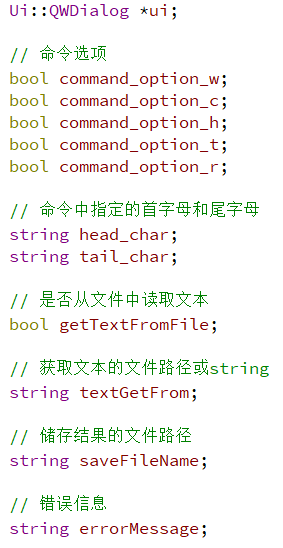### 界面模块与计算模块的对接

API模块主要由两部分组成，第一部分是文件读写部分，第二部分是命令解析部分。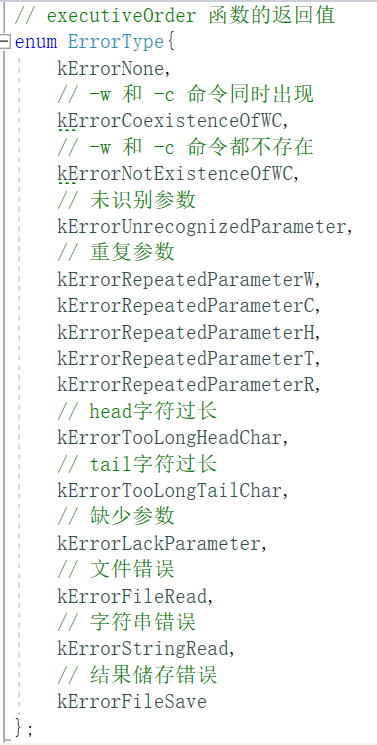executiveCommand函数运行逻辑如下：

CLI：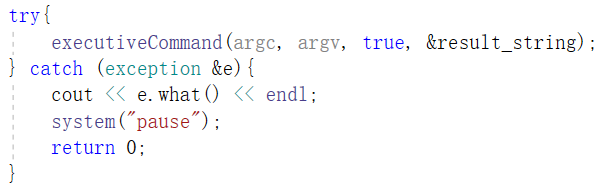GUI：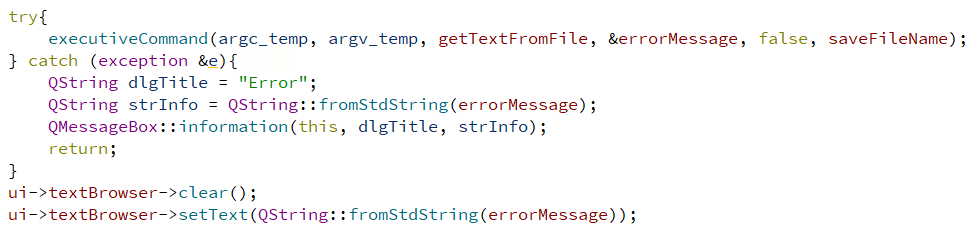### 说明结对编程的优点和缺点。结对的每一个人的优点和缺点在哪里 (要列出至少三个优点和一个缺点)。

• 能够及时完成，积极交流。
• 想法独特，在我向他抱怨单词量太大可能有问题时，他提议针对26个字母单独判断。
• 认真负责。

• 可能对我的督促力度还是不够，我可能在一定程度上拖慢了进度。

### 模块松耦合### 作者的其它热门文章

0
0 收藏

0 评论
0 收藏
0